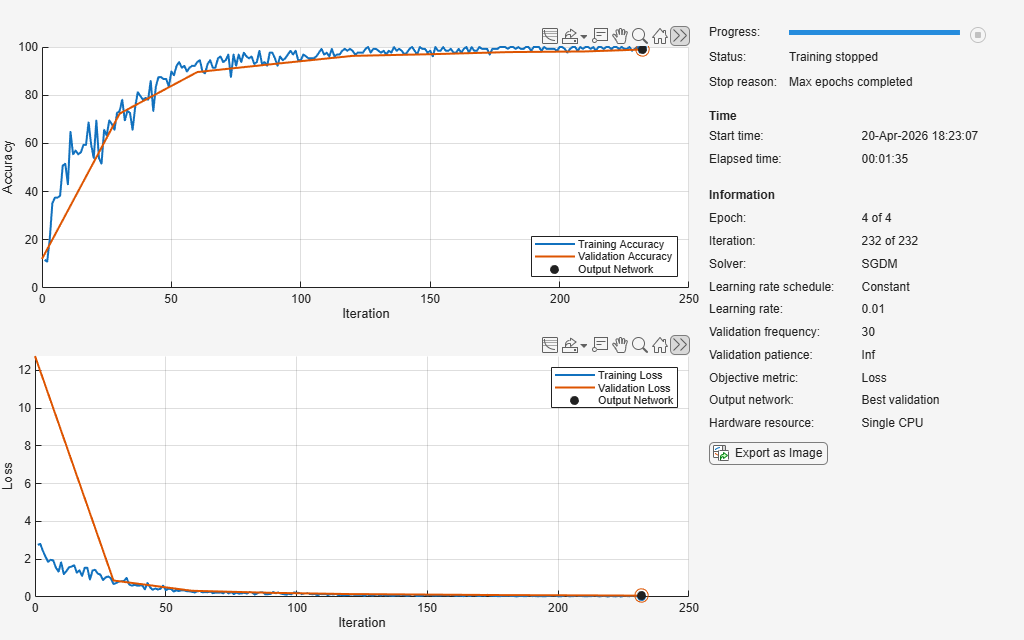# Create Simple Image Classification Network

This example shows how to create and train a simple convolutional neural network for deep learning classification. Convolutional neural networks are essential tools for deep learning and are especially suited for image recognition.

The example demonstrates how to:

• Define the network architecture.

• Specify training options.

• Train the network.

• Predict the labels of new data and calculate the classification accuracy.

For an example showing how to interactively create and train a simple image classification network, see Create Simple Image Classification Network Using Deep Network Designer.

Load the digit sample data as an image datastore. The `imageDatastore` function automatically labels the images based on folder names.

```digitDatasetPath = fullfile(matlabroot,'toolbox','nnet','nndemos', ... 'nndatasets','DigitDataset'); imds = imageDatastore(digitDatasetPath, ... 'IncludeSubfolders',true, ... 'LabelSource','foldernames');```

Divide the data into training and validation data sets, so that each category in the training set contains 750 images, and the validation set contains the remaining images from each label. `splitEachLabel` splits the image datastore into two new datastores for training and validation.

```numTrainFiles = 750; [imdsTrain,imdsValidation] = splitEachLabel(imds,numTrainFiles,'randomized');```

### Define Network Architecture

Define the convolutional neural network architecture. Specify the size of the images in the input layer of the network and the number of classes in the fully connected layer before the classification layer. Each image is 28-by-28-by-1 pixels and there are 10 classes.

```inputSize = [28 28 1]; numClasses = 10; layers = [ imageInputLayer(inputSize) convolution2dLayer(5,20) batchNormalizationLayer reluLayer fullyConnectedLayer(numClasses) softmaxLayer classificationLayer];```

### Train Network

Specify the training options and train the network.

By default, `trainNetwork` uses a GPU if one is available, otherwise, it uses a CPU. Training on a GPU requires Parallel Computing Toolbox™ and a supported GPU device. For information on supported devices, see GPU Computing Requirements (Parallel Computing Toolbox). You can also specify the execution environment by using the `'ExecutionEnvironment'` name-value pair argument of `trainingOptions`.

```options = trainingOptions('sgdm', ... 'MaxEpochs',4, ... 'ValidationData',imdsValidation, ... 'ValidationFrequency',30, ... 'Verbose',false, ... 'Plots','training-progress'); net = trainNetwork(imdsTrain,layers,options);``````YPred = classify(net,imdsValidation); YValidation = imdsValidation.Labels; accuracy = mean(YPred == YValidation)```
```accuracy = 0.9892 ```